MathScore EduFighter is one of the best math games on the Internet today. You can start playing for free!

## Quadratic Graph Zeros - Sample Math Practice Problems

The math problems below can be generated by MathScore.com, a math practice program for schools and individual families. References to complexity and mode refer to the overall difficulty of the problems as they appear in the main program. In the main program, all problems are automatically graded and the difficulty adapts dynamically based on performance. Answers to these sample questions appear at the bottom of the page. This page does not grade your responses.

See some of our other supported math practice problems.

### Complexity=1, Mode=simple

Find the zeros (roots) of the following functions. Whenever there are multiple answers, separate them with commas. Example: 1,3

 1.   y = x 2 - 8x + 15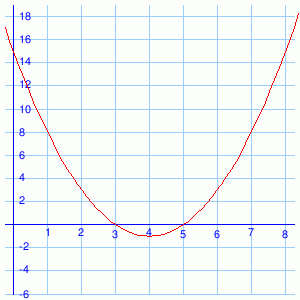x = 2.   y = x 2 - 1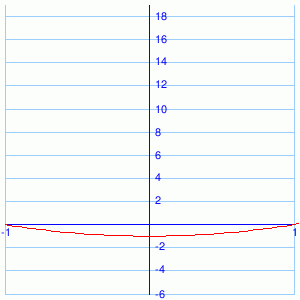x =

### Complexity=3, Mode=prefix

Find the zeros (roots) of the following functions. Whenever there are multiple answers, separate them with commas. Example: 1,3

 1.   y = x 2 + x - 6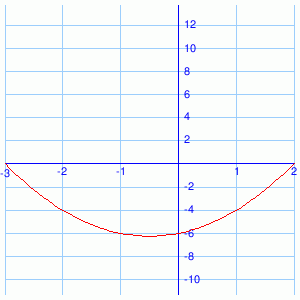x = 2.   y = x 2 - 2x + 1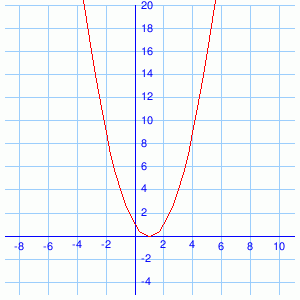x =

### Complexity=4, Mode=prefix

Find the zeros (roots) of the following functions. Whenever there are multiple answers, separate them with commas. Example: 1,3

 1.   y = x 2 + 7x - 44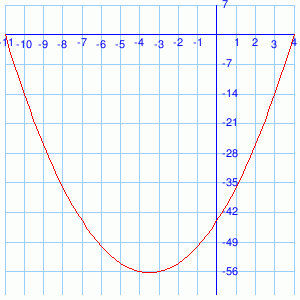x = 2.   y = x 2 + 23x + 42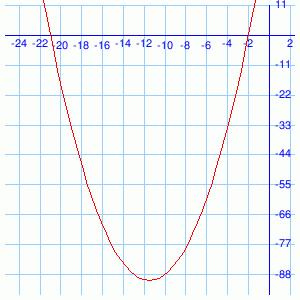x =

### Complexity=1, Mode=simple

Find the zeros (roots) of the following functions. Whenever there are multiple answers, separate them with commas. Example: 1,3

1y = x 2 - 8x + 15x =
Solution
Zeros or roots of a function are just the x-values that make y equal to 0. "Eyeball" the graph to get the answer(s).
You can check the solution by rewriting the equation as y = (x - ?)(x - ?) and see if these values fit. In this case, y = (x - 3) (x - 5).
2y = x 2 - 1x =
Solution
Zeros or roots of a function are just the x-values that make y equal to 0. "Eyeball" the graph to get the answer(s).
You can check the solution by rewriting the equation as y = (x - ?)(x - ?) and see if these values fit. In this case, y = (x - -1) (x - 1).
From the graph, you might have noticed that the parabola is symmetric across the central y-axis. This corresponds to the fact that, in the equation, +/- the same x give the same y. The answers reflect this symmetry.

### Complexity=3, Mode=prefix

Find the zeros (roots) of the following functions. Whenever there are multiple answers, separate them with commas. Example: 1,3

1y = x 2 + x - 6x =
Solution
Zeros or roots of a function are just the x-values that make y equal to 0. "Eyeball" the graph to get the answer(s).
You can check the solution by rewriting the equation as y = (x - ?)(x - ?) and see if these values fit. In this case, y = (x - -3) (x - 2).
2y = x 2 - 2x + 1x =
Solution
Zeros or roots of a function are just the x-values that make y equal to 0. "Eyeball" the graph to get the answer(s).
You can check the solution by rewriting the equation as y = (x - ?)(x - ?) and see if these values fit. In this case, y = (x - 1)2.

### Complexity=4, Mode=prefix

Find the zeros (roots) of the following functions. Whenever there are multiple answers, separate them with commas. Example: 1,3

1y = x 2 + 7x - 44x =
Solution
Zeros or roots of a function are just the x-values that make y equal to 0. "Eyeball" the graph to get the answer(s).
You can check the solution by rewriting the equation as y = (x - ?)(x - ?) and see if these values fit. In this case, y = (x - -11) (x - 4).